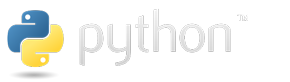# Introduction to Computational General Equilibrium (CGE) Models¶

In this notebook we'll cover some basic ideas that will introduce you to CGE modelling, i.e., of solving Walrasian GE models in a computer. To make things simple we will focus on the easiest CGE model possible: a pure endowment economy with $M$ individuals and $N$ commodities. When $M=2$ and $N=2$, this is an economy that can be represented in an Edgeworth box.

# Set-up our environment as before¶

Let's import the packages we will use and set the paths for outputs.

# Set-up our environment as before¶

Let's import the packages we will use and set the paths for outputs.

# Working withinYou can work with a Jupyter notebook using Stata just as you would using Python. Text and math can be written in Markdown as we have done in the other notebooks. So, use your usual Stata code in code cells, produce figures etc., as if you were in the Stata

#in aNotebook

You can work with Stata in a Python notebook by using the package ipystata. Just like r2py, which allows us to use R in Python, we can now use both (or if you want all three!) programming languages in one notebook.

# Setup¶

Let's start by importing all the packages we want to use.

# Introduction to Dynamic Programming¶

We have studied the theory of dynamic programming in discrete time under certainty. Let's review what we know so far, so that we can start thinking about how to take to the computer.

# The Problem¶

We want to find a sequence $\{x_t\}_{t=0}^\infty$ and a function $V^*:X\to\mathbb{R}$ such that

$$V^{\ast}\left(x_{0}\right)=\sup\limits _{\left\{ x_{t}\right\} _{t=0}^{\infty}}\sum\limits _{t=0}^{\infty}\beta^{t}U(x_{t},x_{t+1})$$

# Faster Computations with Numba¶

## Some notes mostly for myself, but could be useful to you¶

Altough Python is fast compared to other high-level languages, it still is not as fast as C, C++ or Fortran. Luckily, two open source projects Numba and Cython can be used to speed-up computations. Numba is sponsored by the producer of Anaconda

# Working with Economic data in Python¶

This notebook will introduce you to working with data in Python. You will use packages like Numpy to manipulate, work and do computations with arrays, matrices, and such, and anipulate data (see my Introduction to Python). But given the needs of economists (and other scientists) it will be advantageous for us to use pandas

# Using Geographic Information Systems (GIS) inand¶

## Geographic Information Systems (GIS)¶

GIS refers to methods of storing, displaying and analyzing geogaphical information. These methods have become essential in economic analysis (as you have noticed from the reading list for our Ph.D. course on economic growth). For this reason, it is good that you acquaint yourself with these methods. They will prove very useful when doing research, especially to show the spatial distribution of your variables of interest, contructing new measures, or doing spatial analysis.

# Introduction toandusingPython is a powerful and easy to use programming language. It has a large community of developers and given its open source nature, you can find many solutions, scripts, and help all over the web. It is easy to learn and code, and faster than other high-level programming languages...and did I mention it is free